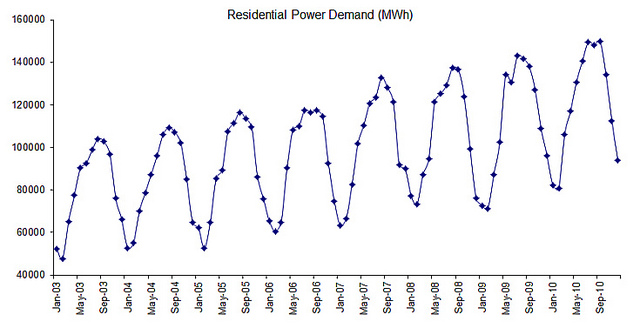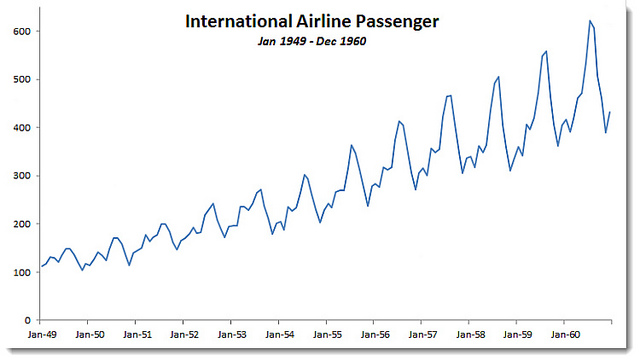# Patterns Unplugged

This is the third issue in our ARMA Unplugged modeling series. In this issue, we introduce the common patterns often found in real-time series data and discuss a few techniques to identify/model those patterns, paving the way for more elaborate discussion decomposition and seasonal adjustment methodologies in future issues.

What are the common patterns, and how do we identify and model them to derive a better understanding of the underlying process, and to better forecast our data? That is the central question for this issue.

## Background

In time series analysis, our main objectives are (a) identifying the nature of the phenomenon represented by the sequence of observations, and (b) forecasting (i.e. predicting) future values of the time series variable.

Once the pattern is established, we can interpret and integrate it with other data (i.e., use it in our theory of the investigated phenomenon, e.g., seasonal commodity prices). Regardless of the depth of our understanding and the validity of our interpretation (theory) of the phenomenon, we can extrapolate the identified pattern to predict future events.

In the majority of time series data, there are two dominant systematic patterns or identifiable components: trend and seasonality.

A Trend is the linear or (more often) non-linear component that changes with time and does not repeat (or at least not within the sample data scope) itself.

Seasonality, on the other hand, repeats itself in a systematic interval over time.

Trend and seasonality may simultaneously exist in real-time data. For example, a company’s sales of a given consumer product may experience variation among the months of the year (e.g. Holiday season), while the sales of that month grow by 10% compared to the sales of the same month in the prior year.

Sound simple? Not really: the presence of irregulars (i.e. noise, shocks, and innovations) makes those components hard to identify.

## Trend Analysis

There are no automatic means of identifying a trend component, but as long as the trend is monotonous (consistent increasing or time series decreasing), a visual examination of the data series plot (or the smoothed version of the data has a lot of noise) can quickly reveal its presence.

In the event the time series exhibits a seasonality component, you can apply a moving average (or median) smoothing function with a window size equal to the length of one period to cancel out noise and seasonality and isolate the trend component.The trend here is assumed to be deterministic; in other words, it follows a fixed relationship with time. The time series may possess a stochastic trend, in which case it can’t be captured with a simple smoothing function. We’ll discuss this later on.

## Seasonality vs. Cycle

Often, we use the term seasonality (or periodicity) when the time series exhibits a pattern that repeats itself. The pattern can be visually detected in the time series plot unless the data has a lot of noise.

In this discussion, we need to make a further distinction in two types of periodicity:

• Deterministic (Seasonality): A seasonal pattern exists when it is influenced by seasonal factors (e.g. quarter of the year, the month, day of the week). Seasonality is fixed and known period. This is why we often call it a “periodic” time series
• Stochastic (Cyclic): a stochastic pattern exists when the data exhibits rises and falls that are not fixed over a period (stochastic). The duration of a period is usually several years, but the duration is not known ahead of time. The business cycle is often used in econometrics literature as an example of a cycle

Going forward, we will assume we mean a deterministic, calendar-driven, fixed period type of seasonality whenever the broad term “seasonality is used. “Cycle,” on the other hand, will refer to stochastic periodicity.

Seasonality

Seasonal dependency is defined as a correlational dependency of order k between the i-th component and (i+k) component. If the measurement error is not too large, it can be visible in the data: visually identified in the series as a pattern that repeats every k element.

The exponential smoothing function (e.g. winter’s triple exponential smoothing) is suitable to capture seasonality and deterministic trend, but not a time series with (stochastic) cycles.

Cycle

For stochastic cycles (i.e. periodicity with a non-fixed period or unknown duration), we can use an ARMA(p, q) type of process: with an autoregressive component order (p) greater than one (1) and additional conditions on the parameters’ values to obtain cyclicity.

For example, consider the ARMA (2,q) process:

$$(1-\phi_1 L -\phi_2 L^2)(r_t-\mu)=(1+\sum_{i=1}^q \theta_iL^i)a_t$$

Let:

$$\phi_1\succ 0$$ $$\phi_2\prec 0$$ $$\phi_1^2+2\phi_2 \prec 0$$

Then AR characteristic equation possesses a complex root, and can be represented as follows:

$$\psi=\frac{-\phi_1\pm \sqrt{\phi_1^2+4\phi_2}}{2\phi_2}=\alpha\pm j\omega$$

The ACF plot of this process exhibits exponential damping sine and cosine waves. The average length of the stochastic cycle $k$ is:

$$k=\frac{2\pi}{cos^{-1}\left(\frac{\phi_1}{2\sqrt{-\phi_2}}\right)}$$

### Seasonality and Cyclicity

It is possible to have both cyclic and seasonal behavior in an ARMA-type model: Seasonal ARIMA (aka SARIMA). For the general case, a multiplicative SARIMA $(p,d,q)\times(P,D,Q)_s$ process is defined as follows:

$$\Phi(L^s)\phi(L)(1-L^s)^D(1-L)^d y_t=\Theta(L^2)\theta(L)a_t$$ $$(1-L^s)^D(1-L)^d y_t=\frac{\Theta(L^s)}{\Phi(L^s)}\times \frac{\theta(L)}{\phi(L)}a_t$$

Where

• $s$ is the length of the seasonal period (deterministic)

We’ll cover SARIMA in greater detail in future issues.

Note:

Long-period cyclicity is not handled very well in the ARMA framework. Alternative (nonlinear) models are usually preferred.

## Time Series Decomposition

In general, the time series $\{y_t\}$ can be broken down into two primary systematic components: trend $\{T_t\}$ and seasonality $\{S_t\}$, with everything else lumped under “Irregulars” $\{I_t\}$ (or noise).

The Irregulars (residuals) component $\{I_t\}$ captures all the non-systemic (i.e. deterministic) properties of the time series, so to improve our forecast further we can model $\{I_t\}$ with an ARMA type of model (e.g. regARIMA).

The time series can be modeled as the sum (i.e. additive decomposition) or the product (multiplicative decomposition) of its components.

In some time series, the amplitude of both the seasonal and irregular variations do not change as the level of the trend rises or falls. In such cases, an additive model is appropriate.

Let’s examine the formulation:

$$y_t=T_t+S_t+I_t$$

To remove the seasonal effect (seasonal adjustment) from the time series:

$$\textrm{SA}_t=y_t-\hat S_t=T_t+I_t$$

Where

• $T_t$ is the trend component
• $S_t$ is the estimated seasonality component

Note:

Under this model, the three components ($S_t,T_t,I_t$) have the same units as the original series.

Multiplicative Decomposition

In many time series, the amplitude of both the seasonal and irregular variations increase as the level of the trend rises. In this situation, a multiplicative model is usually appropriate.

$$y_t=S_t\times T_t\times I_t$$

To remove the seasonal effect from the data:

$$\textrm{SA}_t=\frac{y_t}{\hat S_t}=T_t\times I_t$$Note:

Under this model, the trend has the same units as the original series, but the seasonal and irregular components are unitless factors, distributed (centered) around 1.

The multiplicative model cannot be used when the original time series contains very small or zero values. This is because it is not possible to divide a number by zero. In these cases, a pseudo-additive model combining the elements of both the additive and multiplicative models is used.

$$y_t=T_t+T_t\times (S_t-1)+T_t\times(I_t-1)=T_t\times (S+t+I_t-1)$$

Note:

Under this model, the trend has the same units as the original series, but the seasonal and irregular components are unitless factors, distributed (centered) around 1.

This model assumes that seasonal and irregular variations are both dependent on the level of the trend but independent of each other.

The seasonal adjusted series is defined as follows:

$$\textrm{SA}_t=y_t-\hat T_t (\hat S_t-1)$$

Where

• $T_t$ is the estimated trend component
• $S_t$ is the estimated seasonality component

## Conclusion

In real time-series data, the two primary patterns often observed are trend and seasonality. Furthermore, the time-series data include a noise or error term which lumps all non-systematic factors together and as a result makes the identification of those components a bit difficult. A smoothing or filtering procedure may be required to prepare the data for analysis.

Seasonality is described as a repeated deterministic pattern (i.e. ups and downs) that is driven primarily by calendar-related factors (e.g. day of week, month of the year, holiday, etc.). Cyclicity, on the other hand, is stochastic in nature and does not have a fixed or known period.

In short, a given time series can be viewed as a composition of one or more primitive series (systematic (i.e. deterministic) or stochastic).

There are two distinct types of decomposition models: additive and multiplicative. The decision of which model to use depends on the amplitude of both the seasonal and irregular variations. If they do not change as the level of the trend rises, then additive decomposition is in order; otherwise, a multiplicative one is used. Multiplicative decomposition is found in the majority of time series.

This issue is intended to serve as a preliminary exercise for time series decomposition and time series seasonal adjustment.